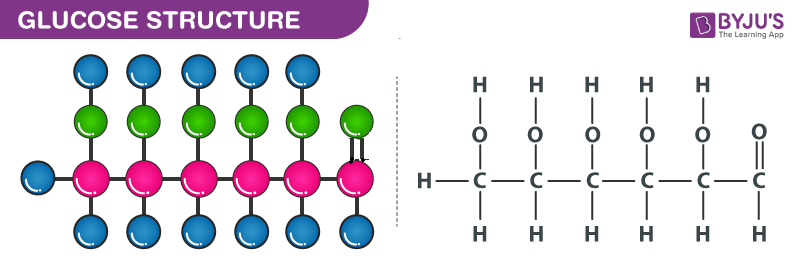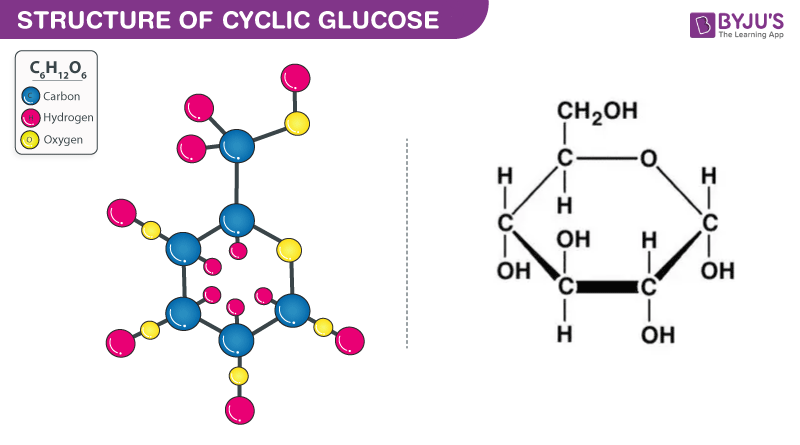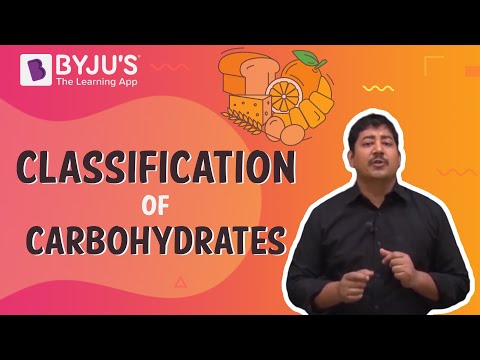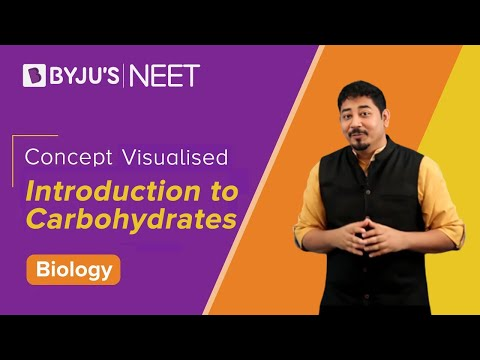Jet Set Go! All about Aeroplanes Jet Set Go! All about Aeroplanes

# Chemical Formula for Glucose Questions

Glucose is a monosaccharide containing six carbon atoms, five hydroxyl groups and one aldehyde group. Its chemical formula is C6H12O6. It is also known as aldohexose and dextrose and exists as a straight chain or ring. It is a vital diet component used to treat hypoglycemia and hyperkalemia.

 Definition: Glucose is a monosaccharide with six carbon atoms, five hydroxyl groups and one aldehyde group. Its chemical formula is C6H12O6.

## Chemical Formula for Glucose Chemistry Questions with Solutions

Q1. What is the chemical formula of glucose?

(a) C5H10O5

(b) C6H12O6

(c) C7H14O7

(d) None of the above

Q2. What is another name for glucose?

(a) Aldohexose

(b) Dextrose

(c) Both (a) and (b)

(d) None of the above

Answer: (c) Both (a) and (b)

Q3. Glucose is soluble in ______ and _______.

(a) Water and acetic acid

(b) Benzene and acetic acid

(c) n-Hexane and acetic acid

(d) All of the above

Answer: (a) Water and acetic acid

Q4. Which of the following functional group is present in glucose?

(a) Aldehyde

(b) Ketone

(c) Both (a) and (b)

(d) None of the above

Answer: (c) Both (a) and (b)

Q5. How many aldehydic groups are there in glucose?

(a) One

(b) Five

(c) Both (a) and (b)

(d) None of the above

Q6. How many alcoholic groups are there in glucose?

(a) One

(b) Five

(c) Both (a) and (b)

(d) None of the above

Q7. Draw the structure of glucose.Q8. Draw the cyclic structure of glucose.Q9. How is glucose prepared from carbohydrates?

Answer: Glucose can be synthesised by hydrolysis of carbohydrates like sucrose, lactose, cellulose, maltose, or glycogen.

C12H22O11 (Sucrose) + H2O → C6H12O6 (Glucose) + C6H12O6 (Fructose)

Q10. How is glucose prepared from starch?

Answer: We can prepare glucose by boiling starch with dilute sulphuric acid at 393 K and 2 to 3 atmosphere pressure.

(C6H12O5)n (Starch) + n H2O → n C6H12O6 (Glucose)

Q11. How can you justify the presence of six carbon atoms in straight-chain glucose?

Answer: We can react straight-chain glucose with hydrogen iodide and red phosphorous to justify the presence of six carbon atoms in straight-chain glucose.

Q12. What is reducing sugar?

Answer: A carbohydrate containing that has free aldehyde or ketone group and gives positive tollens, or fehlling reagent test is referred to as reducing sugar.

Q13. What is the general formula for carbohydrates?

Answer: The general formula for carbohydrates is Cn (H2O)n.

Q14. How can you justify the absence of the aldehyde group in the pentaacetate of D-glucose?

Answer: Glucose reacts with hydroxylamine to form an oxine, whereas the penta acetate of glucose does not react with hydroxyl amine, indicating the absence of a free aldehyde group.

Q15. What are the applications of glucose?

Answer: Glucose is a monosaccharide with six carbon atoms, five hydroxyl groups and one aldehyde group. There are a plethora of applications of glucose.

1. It is used to treat hypoglycemia.

2. It is used as a forerunner for the synthesis.

3. It is used to treat hyperkalemia.

## Practice Questions on Chemical formula for Glucose

Q1. Glucose and fructose differ in ______.

(a) Optical rotation

(b) Taste

(c) Both (a) and (b)

(d) None of the above

Q2. What are biomolecules?

Q3. How can you justify the presence of an aldehyde group in the glucose molecules?

Q4. How can you justify the presence of five hydroxyl groups in the glucose molecules?

Q5. What does the body do when there is no glucose?

Click the PDF to check the answers for Practice Questions.

## Classification Of Carbohydrates And Its Structure## Biomolecules: Introduction to Carbohydrates (Ch-9)